# KVPY-SX 2016 Chemistry Paper with Solutions

KVPY-SX 2016 Chemistry question paper with solutions is a perfect study material for those who are preparing to appear for KVPY examination. Students can download the question paper along with accurate answers and use it to study for the exams. This will help them to learn and revise the important topics and questions as well as understand how the questions are framed, difficulty level, weightage of marks. Additionally, they will be able to significantly improve their problem-solving skills. Students can either view or instantly download the solutions in PDF format for free.

### KVPY SX 2016 - Chemistry

Question 1. The shape of SCl4 is best described as a

1. a) Square
2. b) tetrahedron
3. c) square pyramid
4. d) see-saw

Solution:

SCl4

$S \rightarrow \frac{1s^{2}}{2} \frac{2s^{2}2p^{2}}{8} \frac{3s^{2}3p^{4}}{6}$

$Hybridization = \frac{Valence\, electron\, of\, central\, atom \pm charge + number\, of\, single\, bonded\, atom}{ 2}$

$\frac{6+0+4}{2} = 5 (sp^{3}d)$

5 = lp + BP

⇒ lp = 5 – BP = 5 – 4 = 1

Hence, the shape is K shape or see-saw shape.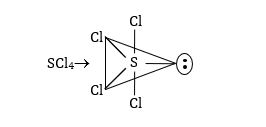Therefore, the correct option is (d).

Question 2. Among the following atomic orbital overlaps, the non-bonding overlap is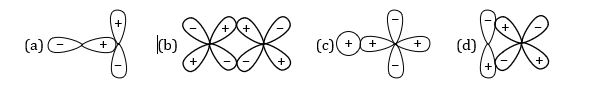Solution:

Overlapping is possible when axis of two orbitals is in same direction but in case of option(A), axis is not in the same direction.

Possible types of overlapping are as follows;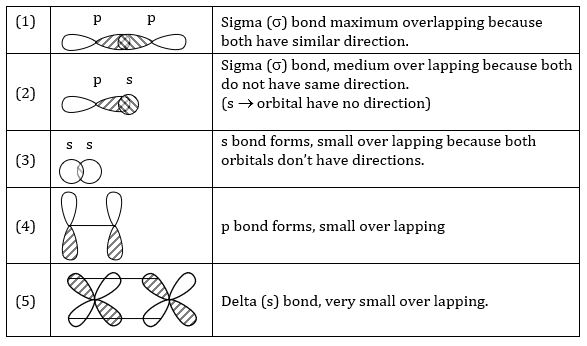Therefore, the correct option is (a).

Question 3. Among the following complexes, the one that can exhibit optical activity is

1. a) [CoCl6]3–
2. b) [Co(en)Cl4]
3. c) cis-[Co(en)2Cl2]+
4. d) trans-[Co(en)2Cl2]+

Solution:

a) All of the ligands are same. Hence, it is not optically active. b) [Co(en)Cl4]Θ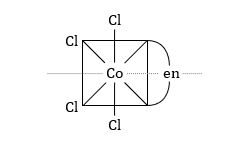Due to the presence of plane of symmetry in elements, it is not optically active.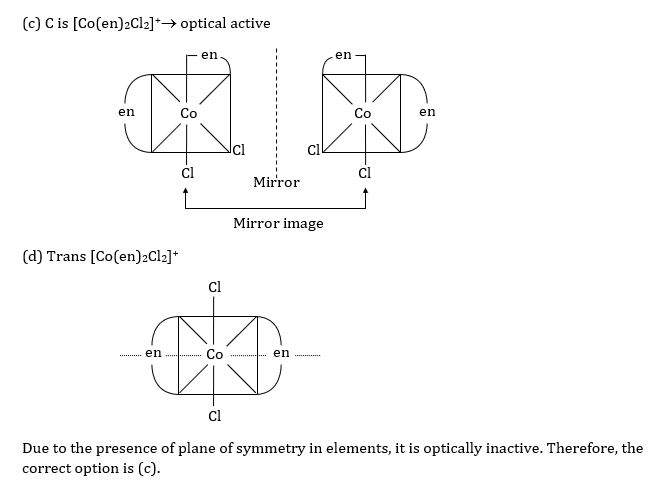Question 4. The pKa of oxoacids of chlorine in water follows the order

1. a)HClO< HClO3< HClO2< HClO4
2. b) HClO4< HClO3< HClO2<HClO
3. c) HClO4< HClO2< HClO3<HClO
4. d) HClO2<HClO< HClO3< HClO4

Solution:

Oxidation state , Acidic strength

[H+] , Acidic strength , K, pKa ↓

 Order of acidic strength HClO4 (+7) > HClO3 (+5) > HClO2 (+3) >HClO (+1)

∴ Order of pKa ⇒ HClO> HClO2> HClO3< HClO4

Therefore, the correct option is (b).

Question 5. The packing efficiency of the face centered cubic (fcc), body centered cubic (bcc) and simple/primitive cubic (pc) lattices follows the order

1. a)fcc> bcc > pc
2. b) bcc >fcc> pc
3. c) pc > bcc >fcc
4. d) bcc > pc >fcc

Solution:

 Unit cell FCC BCC SC Packing efficiency 74% 68% 52.4%

Hence, the order of packing efficiency is FCC > BCC > SC.

Therefore, the correct option is (a).

Question 6. The ratio of root mean square velocity of hydrogen at 50 K to that of nitrogen at 500 K is closest to

1. a) 1.18
2. b) 0.85
3. c) 0.59
4. d) 1.40

Solution:

∵ We know $v_{rms} = \sqrt{\frac{3RT}{M}}$

R → gas constant

M → Molecular weight of gas (g mol–1)

T → Temperature (K)

⇒ M for H2 = 2 g mol–1

T = 50 K

⇒ M for N2 = 28 g mol–1

T = 500 K

$\frac{(v_{rms})_{H_{2}}}{(v_{rms})_{N_{2}}} = \sqrt{\frac{\frac{3\times R\times 50}{2}}{\frac{3\times R\times 50}{28}}} = 1.18$

Therefore, the correct option is (a).

Question 7. The molecule with the highest dipole moment among the following is

1. a) NH3
2. b) NF3
3. c) CO
4. d) HF

Solution:

 Molecule NH3 NF3 CO HF Dipole moment (µ) 1.46 D 0.24 D 0.122 D 1.82 D

• ∆EN , µ  [CO, HF]

• µ , EN of central atom  (NH3)

• µ ↓, EN of surrounding atoms  (NF3)

Therefore, the correct option is (d).

Question 8. The most stable Lewis acid-base adduct among the following is

1. a) H2O → BCl3
2. b) H2S → BCl3
3. c) H3N → BCl3
4. d) H3P → BCl3

Solution:

Greater the tendency to donate lp, more stable will be the Lewis acid base adduct.

Here, B binds especially with nonmetals N and O [due to good overlapping], so option (a) and (c) have this advantage.

But electronegativity of N is less as compared to O.

(Electronegativity  electron-donating tendency ↓)

Hence, here H3N → BCl3 is most stable acid base adduct.

Therefore, the correct option is (c).

Question 9. The reaction of D-glucose with ammoniacal AgNO3 produces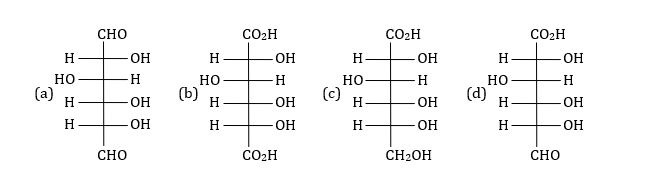Solution: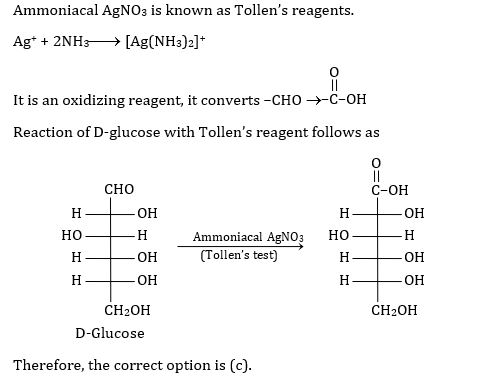Question 10. The reagent (s) used for the conversion of benzene diazonium hydrogen sulfate to benzene is/are -

1. a) H2O
2. b) H3PO2 + H2O
3. c) H2SO4 + H2O
4. d) CuCl/HCl

Solution: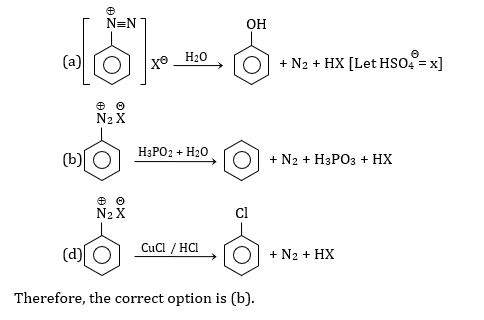Question 11. The major product obtained in the reaction of toluene with 1-bromo-2-methyl propane in the presence of anhydrous AlCl3 is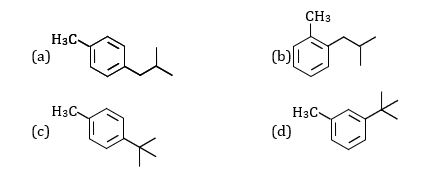Solution:

Reaction of Toluene with 1-Bromo-2-methyl propane follows as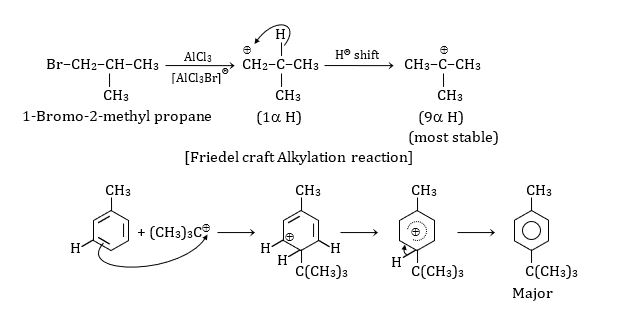Note: Here, both O and P products are possible but due to steric hindrance Ortho is minor and Para is the major product.

Therefore, the correct option is (c).

Question 12. The major product in the following reaction is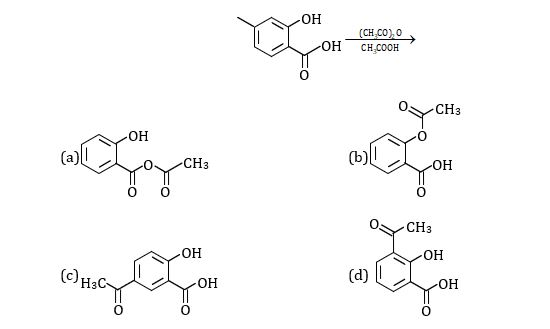Solution:

Acetylation reaction of salicylic acid follows;Therefore, the correct option is (b).

Question 13. The compounds containing sp hybridized carbon atom are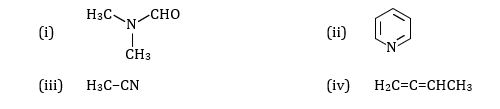1. a) (i) and (ii)
2. b) (iii) and (iv)
3. c) (ii) and (iii)
4. d) (i) and (iv)

Solution: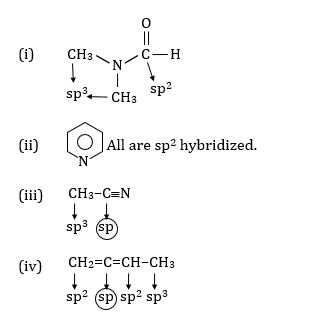Hence, here compound (iii) and (iv) contain ‘sp’ carbon atom.

Therefore, the correct option is (b).

Question 14. Upon heating with acidic KMnO4 an organic compound produces hexan-1,6-dioic acid as the major product. The starting compound is

1. a)Benzene
2. b) cyclohexene
3. c) 1-methylcyclohexene
4. d) 2-methylcyclohexene

Solution: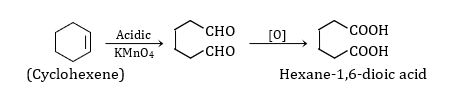Therefore, the correct option is (b).

Question 15. It takes 1h for a first order reaction to go to 50% completion. The total time required for the same reaction to reach 87.5% completion will be

1. a) 1.75 h
2. b) 6.00 h
3. c) 3.50 h
4. d) 3.00 h

Solution:

Given: t½ = 1 hr

∵ We know kt = ln $\left ( \frac{a}{a-x} \right )$ ………(1) {a = initial concentration}

$k = \frac{1}{t} 1n \left ( \frac{a}{a-x} \right ) = \frac{1}{1}1n\left ( \frac{a}{a/2} \right )$ {at t½ = a/2}

$k = \frac{1}{1}1n(2)$

Time required for 87.5% completion = ?

Now, from equation (1)

$t= \frac{1}{1n(2)} \left ( \frac{a}{a-0.875a} \right )$

$t= \frac{1}{1n(2)} \left ( \frac{a}{a/8} \right )$ {t = time, k = rate constant}

$t= \frac{1n8}{1n2} = \frac{2.079}{0.693} = 3hrs$

Therefore, the correct option is (d).

Question 16. A unit cell of calcium fluoride has four calcium ions. The number of fluoride ions in the unit cell is

1. a) 2
2. b) 4
3. c) 6
4. d) 8

Solution:

Formula of calcium fluoride: CaF2→ Ca2+ + 2F

Number of Ca2+ ion in unit cell = 4

So, to maintain electrical neutrality, the number of F present in unit cell

Let the oxidation number of F = x {Oxidation number of Ca = +2 and F = –1}

Then, 4(+2) + x(–1) = 0

x = 8

So, the number of F ion in unit cell = 8

Therefore, the correct option is (d).

Question 17. The equilibrium constant of a 2-electron redox reaction at 298 K is 3.8 × 10–3. The cell potential E° (in V) and the free energy change ∆G° (in kJ mol–1) for this equilibrium respectively, are

1. a) –0.071, –13.8
2. b) –0.071, 13.8
3. c) 0.71, –13.8
4. d) 0.071, –13.8

Solution:

Given equilibrium constant (K) = 3.8 × 10–3

Temperature = 298 K

Number of electron (n) = 2

∵ We know that ∆ G = –2.303RTlogK = –nF E°cell

∆G = –2.303 × 8.314 × 298 × log(3.8 × 10–3)

⇒∆G = 13809.387 J = 13.809 kJ

∆G = –nF E°cell

13809.387 = –2 × 96500 × E°cell

⇒E°cell = –0.071 V

Therefore, the correct option is (b).

Question 18. The number of stereoisomer possible for the following compound is CH3–CH=CH–CH(Br)–CH2–CH3

1. a) 2
2. b) 3
3. c) 4
4. d) 8

Solution: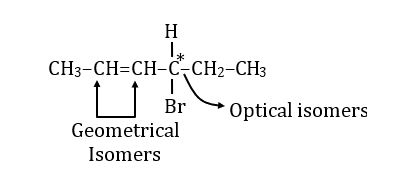n = 2 (Number of steriogenic-centre)

Total stereoisomers = 2n (when symmetry absent)

= 22 = 4

Therefore, the correct option is (c).

Question 19. In the radioactive disintegration series $_{232}^{90}\textrm{Th}\rightarrow _{208}^{82}\textrm{Pb}$, involving α and β decay, the total number of α and β particles emitted are

1. a) 6 α and 6 β
2. b) 6 α and 4 β
3. c) 6 α and 5 β
4. d) 5 α and 6 β

Solution:

Let, the numbers of α and β particles involved are n1and n2 respectively.

90Th232→ 82Pb208 + n1α + n2β {α → [2He4], β→ –1e0}

α-decay

PXA→ P–2XA–4 + 2He4

β-decay

PXA→ P+1XA + –1e0

Mass Balance

⇒ Mass of α particle = 4

232 = 208 + n1 × 4

⇒ n1 = 6

Balancing of proton and atomic number

90 = 82 + 2n1 – n2

n2 = 4

Therefore, the correct option is (b).

Question 20. In the following compressibility factor (Z) vs pressure graph at 300 K, the compressibility of CH4 at pressure < 200 bar deviates from ideal behavior because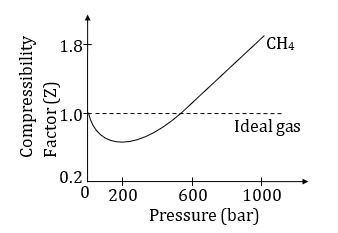1. a) The molar volume of CH4 is less than its molar volume in the ideal state.
2. b) The molar volume of CH4 is same as that in its ideal state.
3. c) Intermolecular interactions between CH4 molecules decrease.
4. d) The molar volume of CH4 is more than its molar volume in the ideal state.

Solution:

Compressibility factor (z) = $\frac{PV}{RT}=\frac{V_{real}}{V_{ideal}}$ …(1)

∵ Z < 1 at P < 200 bar

∴ from equation (1)

$\frac{V_{real}}{V_{ideal}}< 1$

⇒ Vr<Vi

Hence, the molar volume of CH4 is less than its molar volume in the ideal state.

Therefore, the correct option is (a).

Question 21. X, Y and Z in the following reaction sequence are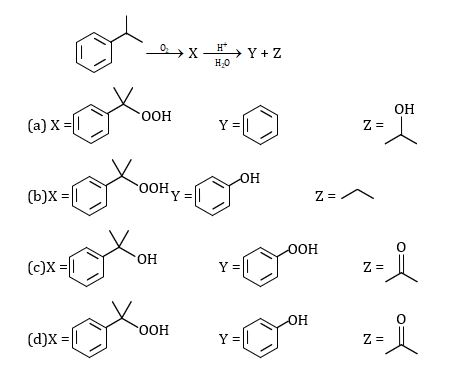Solution: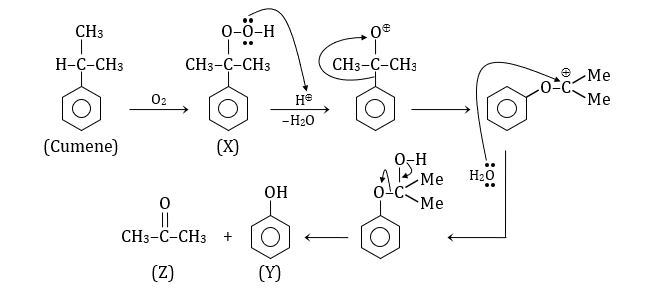Therefore, the correct option is (d).

Question 22. The reagent required for the following two step transformation are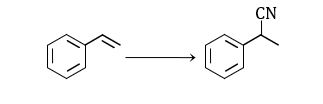1. a) (i) HBr, benzoyl peroxide; (ii) CH3CN
2. b) (i) HBr, (ii) NaCN
3. c) (i) Br2, (ii) NaCN
4. d) (i) NaBr, (ii) NaCN

Solution: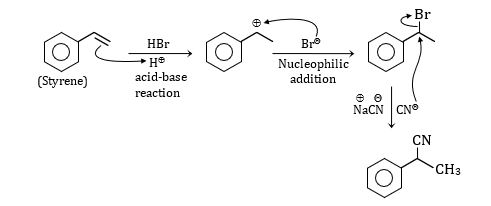Therefore, the correct option is (b).

Question 23. In the reaction sequence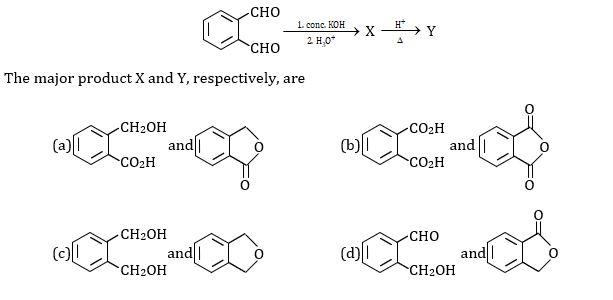Solution: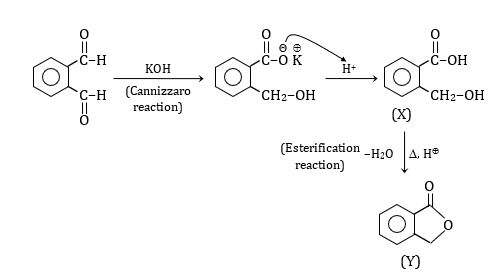Therefore, the correct option is (a).

Question 24. In the following reactions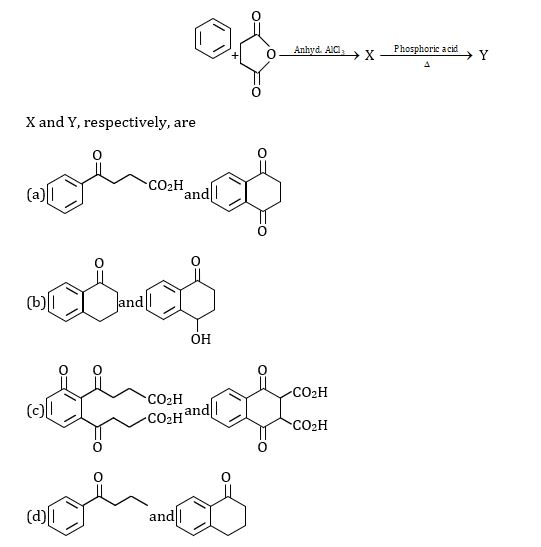Solution: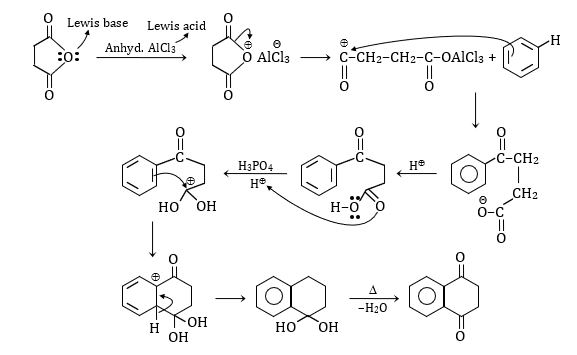Therefore, the correct option is (a).

Question 25. Copper (atomic mass = 63.5) crystallizers in a FCC lattice and has density 8.93 g.cm–3. The radius of copper atom is closest to

1. a) 361.6 pm
2. b) 511.4 pm
3. c) 127.8 pm
4. d) 102.8 pm

Solution:

Given Atomic mass of Cu = 63.5 g

Lattice FCC→ N = 4

d = 8.93 g/cm3

∵ We know that $d = \frac{N\times M}{N_{A}\times a^{3}}$

$\Rightarrow a^{3} = \frac{N\times M}{N_{A}\times d}$

$\Rightarrow a^{3} = \frac{4\times 63.5}{6.023\times 10^{23}\times 8.93}$

a3 = 47.2 × 10–24

⇒ a = (47.2 × 10–24)1/3

a = 3.61 × 10–8 cm

a = 3.61 × 10–10 m

a = 361 × 10–12 m

a = 361 pm

∵ a = r (for FCC)

$r = \frac{a}{2\sqrt{2}} = \frac{361}{2\sqrt{2}} = 127.8 pm$

Therefore, the correct option is (c).

Question 26. Given the standard potentials E°(Cu2+/Cu) and E°(Cu+/Cu) as 0.340 V and 0.522 V respectively, the value of E°(Cu2+/Cu+) is

1. a) 0.364 V
2. b) 0.158 V
3. c) –0.182 V
4. d) –0.316 V

Solution: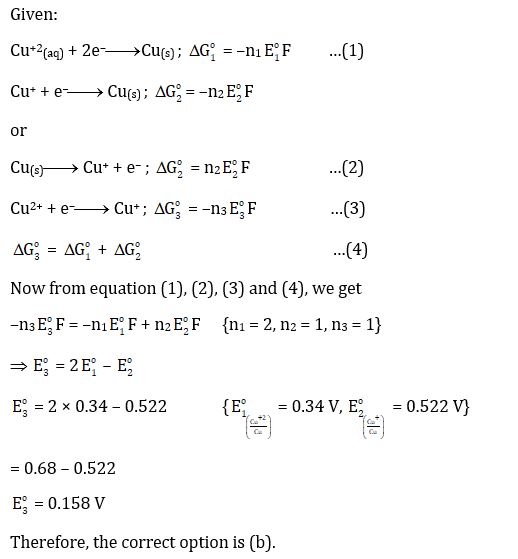Question 27. For electroplating, 1.5 amp current is passed for 250 s through 250 mL of 0.15 M solution of MSO4. Only 85% of the current was utilized for electrolysis. The molarity of MSO4 solution after electrolysis is closest to. [Assume that the volume of the solution remained constant]

1. a) 0.14
2. b) 0.014
3. c) 0.07
4. d) 0.035

Solution:

By Faraday’s law we know that

Equivalent = $r = \frac{I\times t}{96500}$ {where, I = current and t = time}

Equivalent = $\frac{wt.}{eq.wt.}$

Moles (n) = $= \frac{I\times t}{96500\times v.f.}$

∵ According to question only 85% current utilized for electrolysis.

∴ Deposited moles (n) = $\frac{1.5\times 250}{96500\times 2} \times \frac{85}{100} = 0.00135 moles$

$Molarity = \frac{moles\, of\, solute}{volume (L)}$

$0.15 = \frac{n\times 1000}{250}$

⇒ n = 0.375

Hence, the initial moles = 0.375 moles

Deposited moles = 0.00165 moles

Number of moles left in solution = 0.375 – 0.00165 = 0.03585 (moles)

Molarity = $\frac{0.03585\times 1000}{250} = 0.143 M$

Therefore, correct option is (a).

Question 28. The hybridization of the central atom and the shape of [IO2F5]2– ion respectively, are–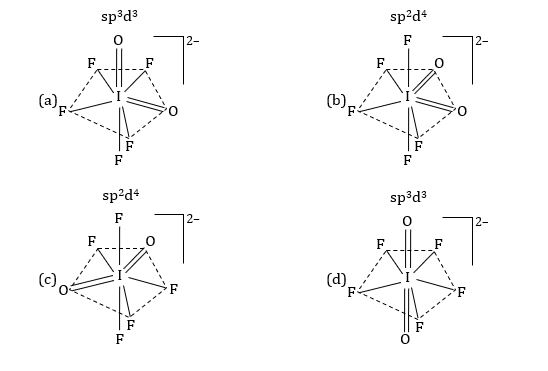Solution:

[IO2F5]2–→ Central atom = I

$Hybridization = \frac{Valence\, electron\, of\, central\, atom \pm charge + number\, of\, single\, bonded\, atom}{ 2}$

Hybridization (n) = $\frac{7+5+2}{2} = 7 (sp^{3}d^{3})$

Hence, the shape is pentagonal bipyramidal.

∵ Double bond causes more repulsion, so they would be on axial position (180° angle to each other).

Therefore, the correct option is (d).

Question 29. 2.33 g of compound X (empirical formula CoH12N4Cl3) upon treatment with excess AgNO3 solution produces 1.435 g of a white precipitate. The primary and secondary valences of cobalt in compound X, respectively, are; [Given: Atomic mass: Co = 59, Cl = 35.5, Ag = 108]

1. a) 3, 6
2. b) 3, 4
3. c) 2, 4
4. d) 4, 3

Solution:

Weight of the compound (x) = 2.33 g

Molecular weight of the compound x, (CoH12N4Cl3)

= 59 + 12 × 1 + 14 × 4 + 35.5 × 3

= 233.5 gmol–1

Moles of compound (x) = $\frac{2.33}{233.5} \approx 0.01\, moles$

Weight of AgCl = 1.435 g

Molecular weight of AgNO3 = 143.5 g mol–1

Moles of AgNO3 = $\frac{1.435}{143.5} = 0.01\, moles$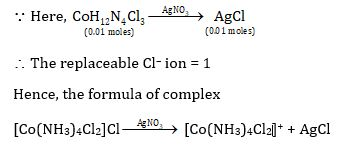Oxidation state of Co in complex = x + 4 × 0 + 2 × (–1)

⇒ x = +3

Coordination number of Co = 6

Hence, the primary valence is 3 and secondary valence is 6.

Therefore, the correct option is (a).

Question 30. The specific conductance (k) of 0.02 M aqueous acetic acid solution at 298 K is 1.65 × 10–4 S cm–1. The degree of dissociation of acetic acid is [Given: equivalent conductance at infinite dilution of H+ = 349.1 S cm2mol–1 and CH3COO = 40.9 S cm2mol–1]

1. a) 0.021
2. b) 0.21
3. c) 0.012
4. d) 0.12

Solution:

Given:

K = 1.65 × 10–4Scm–1

Molarity of CH3COOH = 0.02 M

$\lambda _{H}^{\alpha }$ = 349.1 Scm2mol–1, CHCOO = 40.9 Scm2mol–1

∵ We know $\alpha = \frac{\lambda }{\lambda _{H}^{\alpha }}$ …(1)

$\lambda_{m} = \frac{1000\times k}{M} = \frac{1000\times 1.65\times 10^{-4}}{0.02}$

λm = 8.25 Scm2mol–1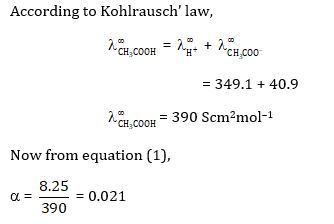Therefore, the correct option is (a).

### KVPY SX 2016 Chemistry Paper with Solutions